This lesson is being piloted (Beta version)

# Plot Raster Data in R

## Overview

Teaching: 40 min
Exercises: 20 min
Questions
• How can I create categorized or customized maps of raster data?

• How can I customize the color scheme of a raster image?

• How can I layer raster data in a single image?

Objectives
• Build customized plots for a single band raster using the `ggplot2` package.

• Layer a raster dataset on top of a hillshade to create an elegant basemap.

## Things You’ll Need To Complete This Episode

See the lesson homepage for detailed information about the software, data, and other prerequisites you will need to work through the examples in this episode.

##The Data

In this lesson, we will be working with two field sites: the Harvard Forest (`HARV`) and San Joaquin Experimental Range (`SJER`).

In this lesson, the raster we will use is: `HARV_dsmCrop.tif`.

For the challenges in this lesson, we will use both and `HARV_dsmCrop.tif` and `HARV_DSMhill.tif`.

We will first start by loading in the raster `DSM_HARV` and converting it to a dataframe again:

``````DSM_HARV <-
raster("data/raster/HARV_dsmCrop.tif")
DSM_HARV_df <- as.data.frame(DSM_HARV, xy = TRUE)
str(DSM_HARV_df)
``````
``````'data.frame':	968044 obs. of  3 variables:
\$ x           : num  731454 731454 731456 731456 731458 ...
\$ y           : num  4713370 4713370 4713370 4713370 4713370 ...
\$ HARV_dsmCrop: num  370 371 371 362 370 ...
``````

## Plot Raster Data in R

This episode covers how to plot a raster in R using the `ggplot2` package with customized coloring schemes. It also covers how to layer a raster on top of a hillshade to produce an eloquent map. We will continue working with the Digital Surface Model (DSM) raster for the NEON Harvard Forest Field Site.

## Plotting Data Using Breaks

In the previous episode, we viewed our data using a continuous color ramp. For clarity and visibility of the plot, we may prefer to view the data “symbolized” or colored according to ranges of values. This is comparable to a “classified” map. To do this, we need to tell `ggplot` how many groups to break our data into, and where those breaks should be. To make these decisions, it is useful to first explore the distribution of the data using a bar plot. To begin with, we will use `dplyr`’s `mutate()` function combined with `cut()` to split the data into 3 bins.

``````DSM_HARV_df <- DSM_HARV_df %>%
mutate(fct_elevation = cut(HARV_dsmCrop, breaks = 3))

ggplot() +
geom_bar(data = DSM_HARV_df, aes(fct_elevation))
``````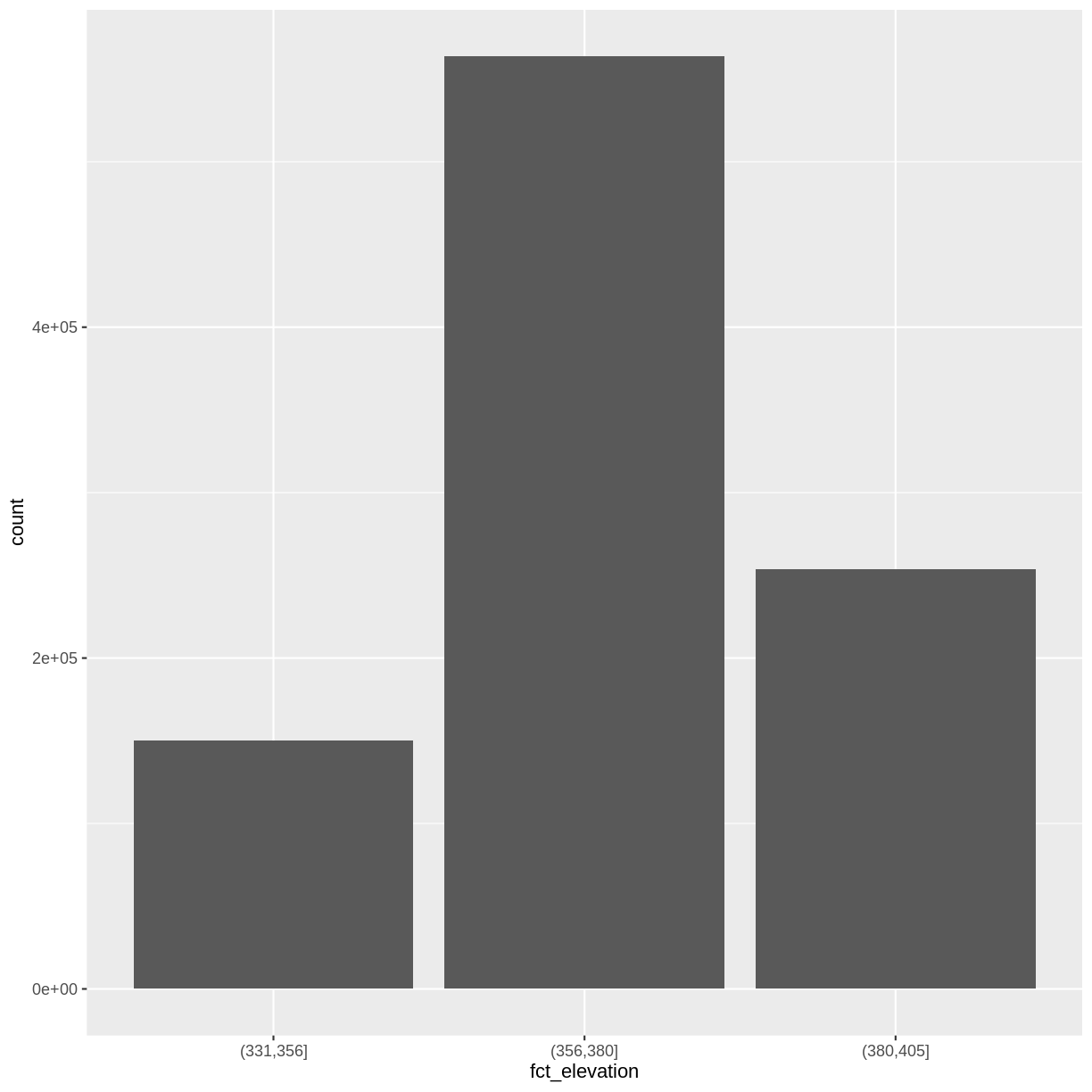If we want to know the cutoff values for the groups, we can ask for the unique values of `fct_elevation`:

``````unique(DSM_HARV_df\$fct_elevation)
``````
`````` (356,380] (380,405] (331,356]
Levels: (331,356] (356,380] (380,405]
``````

And we can get the count of values in each group using `dplyr`’s `group_by()` and `count()` functions:

``````DSM_HARV_df %>%
group_by(fct_elevation) %>%
count()
``````
``````# A tibble: 3 x 2
# Groups:   fct_elevation 
fct_elevation      n
<fct>          <int>
1 (331,356]     150374
2 (356,380]     563694
3 (380,405]     253976
``````

We might prefer to customize the cutoff values for these groups. Lets round the cutoff values so that we have groups for the ranges of 301–350 m, 351–400 m, and 401–450 m. To implement this we will give `mutate()` a numeric vector of break points instead of the number of breaks we want.

``````custom_bins <- c(300, 350, 400, 450)

DSM_HARV_df <- DSM_HARV_df %>%
mutate(fct_elevation_2 = cut(HARV_dsmCrop, breaks = custom_bins))

unique(DSM_HARV_df\$fct_elevation_2)
``````
`````` (350,400] (300,350] (400,450]
Levels: (300,350] (350,400] (400,450]
``````

## Data Tips

Note that when we assign break values a set of 4 values will result in 3 bins of data.

The bin intervals are shown using `(` to mean exclusive and `]` to mean inclusive. For example: `(305, 342]` means “from 306 through 342”.

And now we can plot our bar plot again, using the new groups:

``````ggplot() +
geom_bar(data = DSM_HARV_df, aes(fct_elevation_2))
``````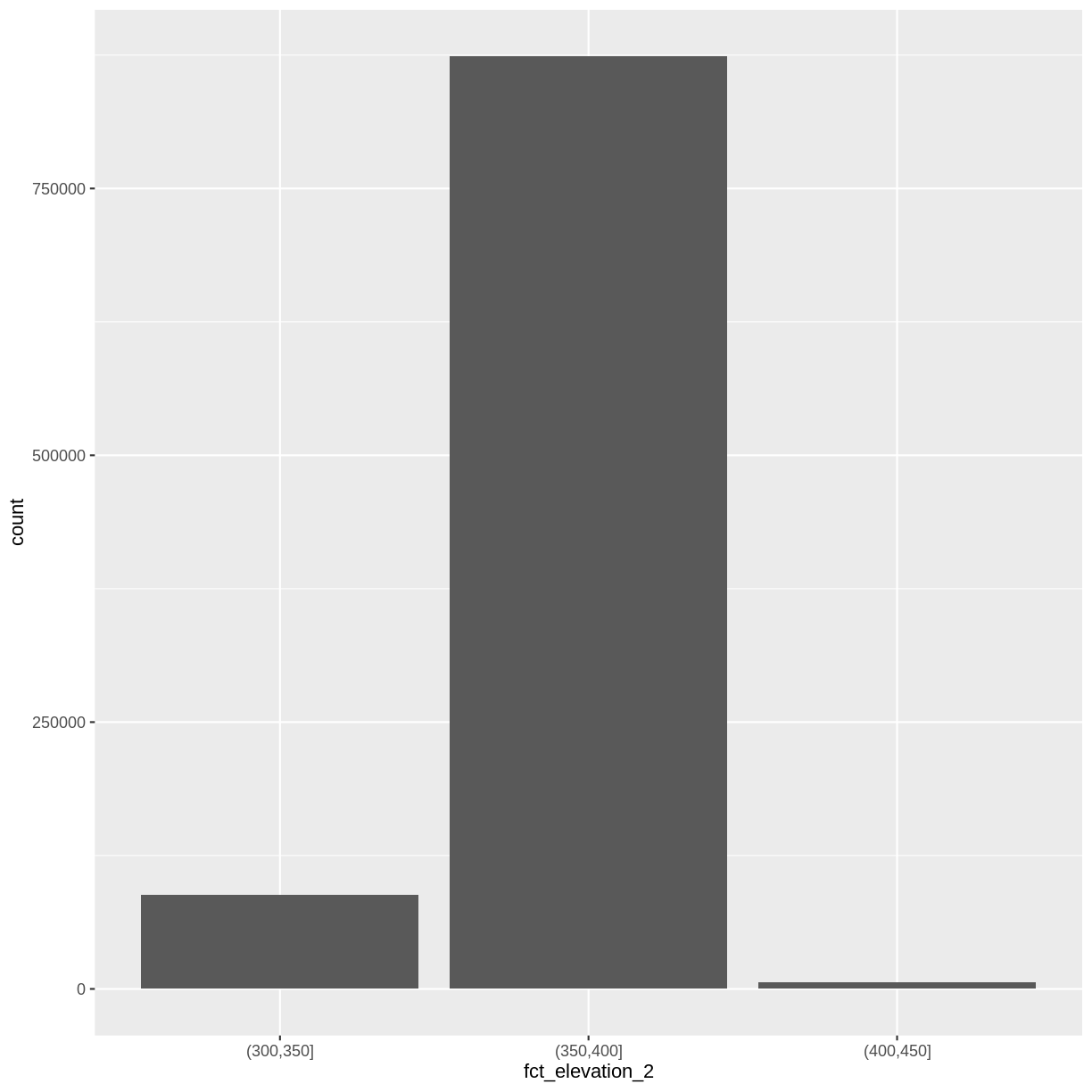And we can get the count of values in each group in the same way we did before:

``````DSM_HARV_df %>%
group_by(fct_elevation_2) %>%
count()
``````
``````# A tibble: 3 x 2
# Groups:   fct_elevation_2 
fct_elevation_2      n
<fct>            <int>
1 (300,350]        87953
2 (350,400]       873767
3 (400,450]         6324
``````

We can use those groups to plot our raster data, with each group being a different color:

``````ggplot() +
geom_raster(data = DSM_HARV_df , aes(x = x, y = y, fill = fct_elevation_2)) +
coord_quickmap()
``````### More Plot Formatting

If we need to create multiple plots using the same color palette, we can create an R object (`my_col`) for the set of colors that we want to use. We can then quickly change the palette across all plots by modifying the `my_col` object, rather than each individual plot. In this example, we will set `my_col` to three terrain colors corresponding to our three groups: 301–350 m, 351–400 m, and 401–450 m.

We can label the x- and y-axes of our plot too using `xlab` and `ylab`. We can also give the legend a more meaningful title by passing a value to the `name` argument of the `scale_fill_manual()` function.

``````my_col <- terrain.colors(3)

ggplot() +
geom_raster(data = DSM_HARV_df , aes(x = x, y = y,
fill = fct_elevation_2)) +
scale_fill_manual(values = my_col, name = "Elevation") +
coord_quickmap()
``````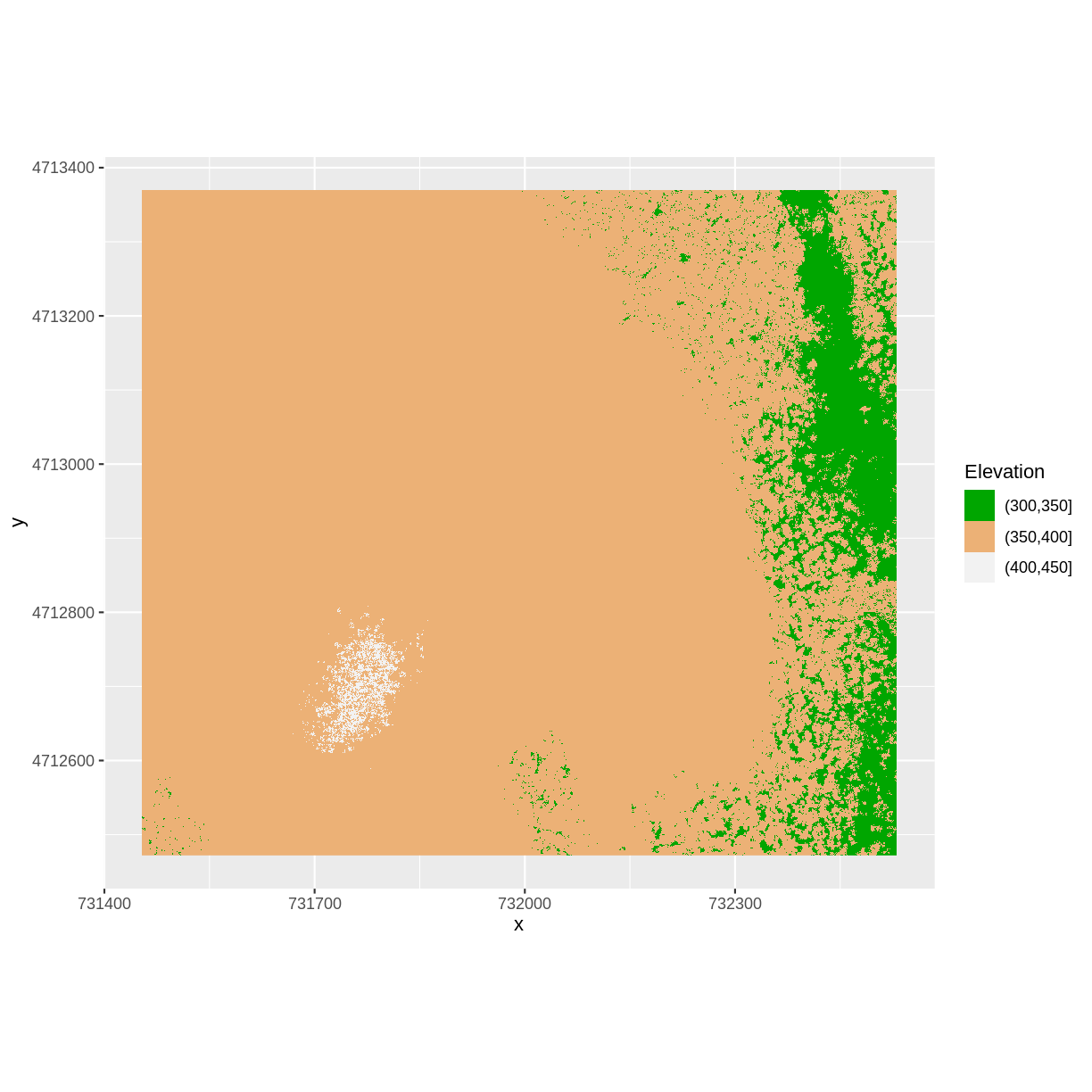We can also modify the x-axis and y-axis labels with the function `xlab()` and `ylab()`:

``````ggplot() +
geom_raster(data = DSM_HARV_df , aes(x = x, y = y,
fill = fct_elevation_2)) +
scale_fill_manual(values = my_col, name = "Elevation") +
xlab("UTM Easting (m)") +
ylab("UTM Northing (m)") +
coord_quickmap()
``````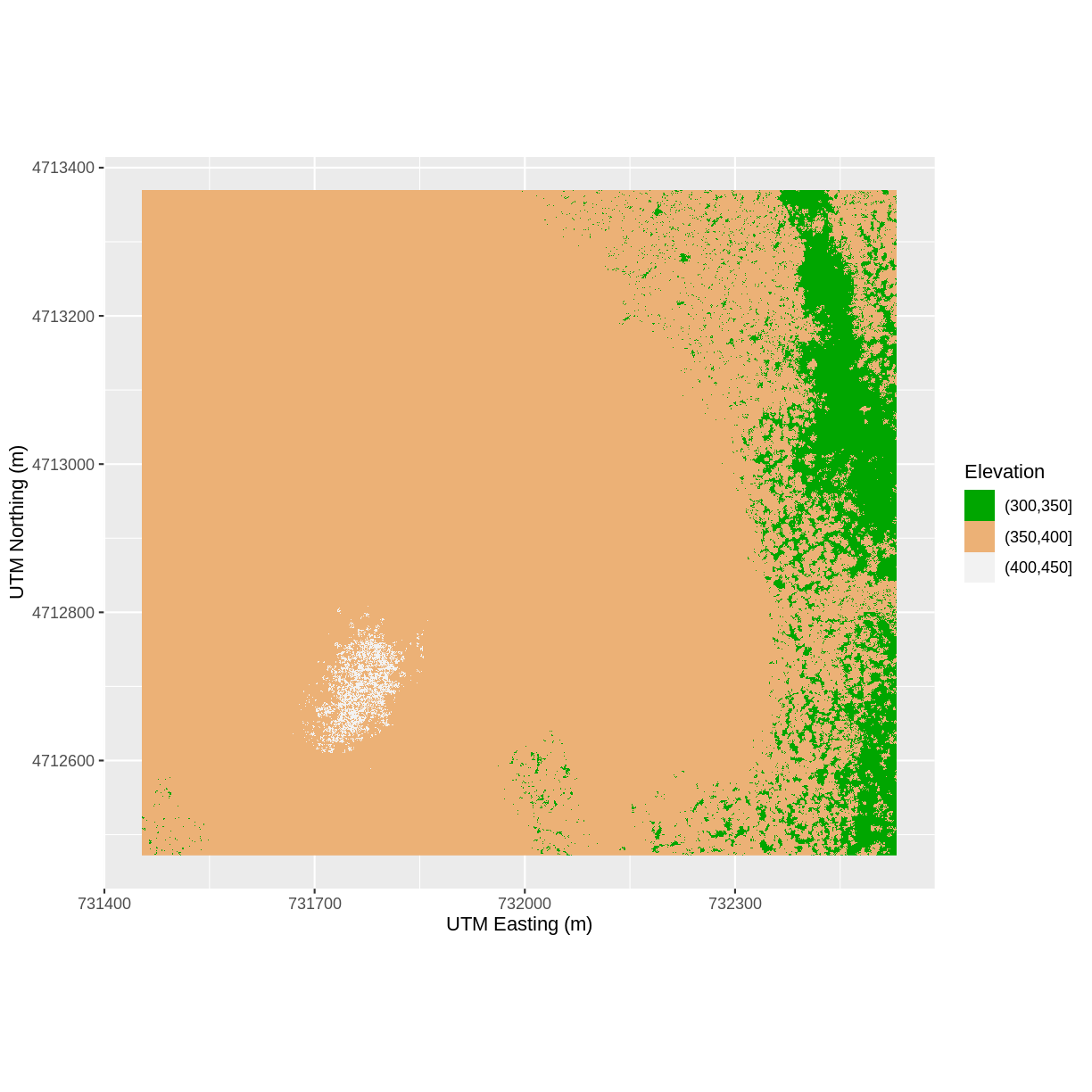## Challenge: Plot Using Custom Breaks

Create a plot of the Harvard Forest Digital Surface Model (DSM) that has:

1. Six classified ranges of values (break points) that are evenly divided among the range of pixel values.
2. Axis labels.
3. A plot title.

## Answers

``````DSM_HARV_df <- DSM_HARV_df  %>%
mutate(fct_elevation_6 = cut(HARV_dsmCrop, breaks = 6))

my_col <- terrain.colors(6)

ggplot() +
geom_raster(data = DSM_HARV_df , aes(x = x, y = y,
fill = fct_elevation_6)) +
scale_fill_manual(values = my_col, name = "Elevation") +
ggtitle("Classified Elevation Map - NEON Harvard Forest Field Site") +
xlab("UTM Easting Coordinate (m)") +
ylab("UTM Northing Coordinate (m)") +
coord_quickmap()
``````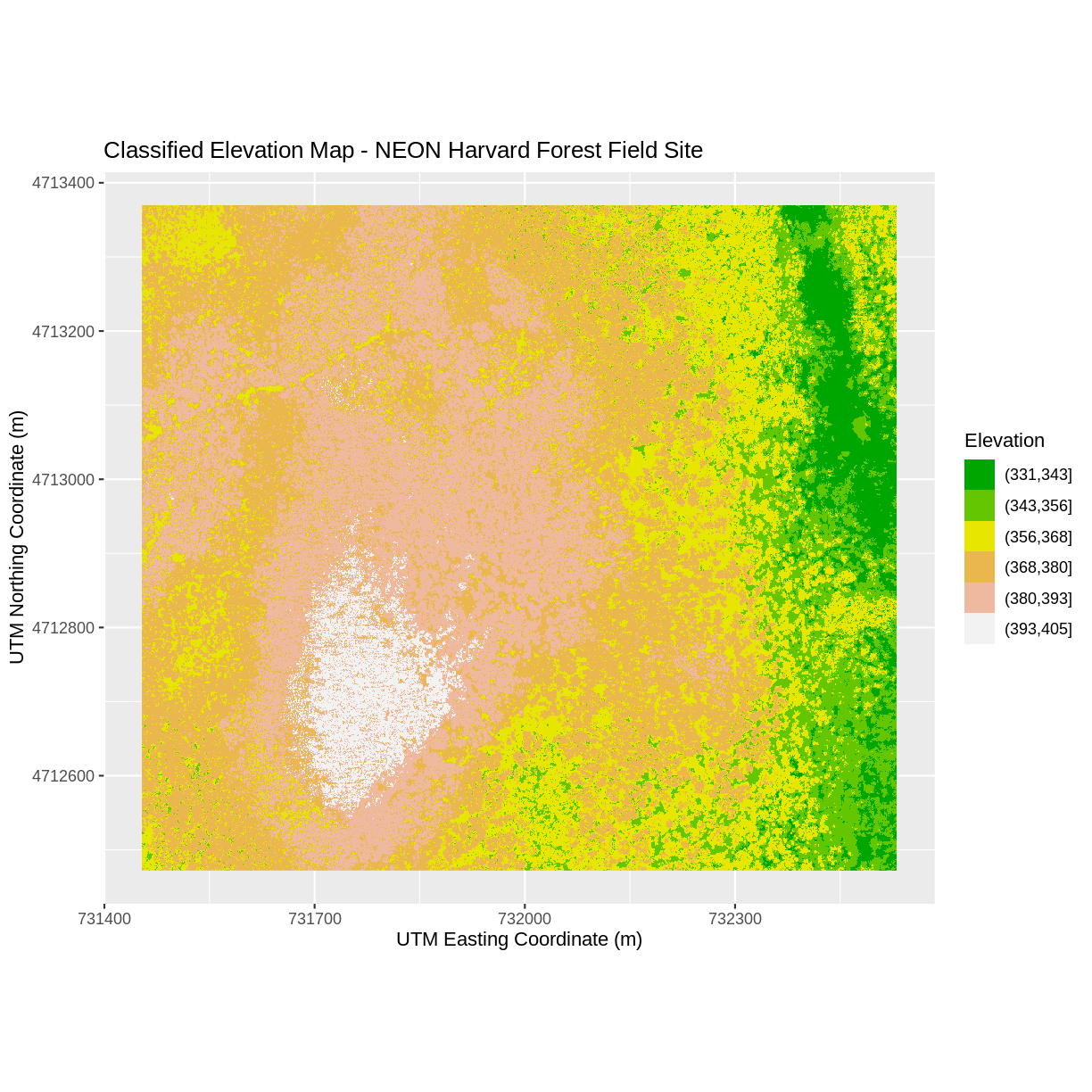## Layering Rasters

We can layer a raster on top of a hillshade raster for the same area, and use a transparency factor to create a 3-dimensional shaded effect. A hillshade is a raster that maps the shadows and texture that you would see from above when viewing terrain. We will add a custom color, making the plot grey.

First we need to read in our DSM hillshade data and view the structure:

``````DSM_hill_HARV <-
raster("data/raster/HARV_DSMhill.tif")

DSM_hill_HARV
``````
``````class      : RasterLayer
dimensions : 898, 1078, 968044  (nrow, ncol, ncell)
resolution : 1, 1  (x, y)
extent     : 731453, 732531, 4712472, 4713370  (xmin, xmax, ymin, ymax)
crs        : +proj=utm +zone=18 +datum=WGS84 +units=m +no_defs +ellps=WGS84 +towgs84=0,0,0
source     : /home/travis/build/UW-Madison-DataScience/r-raster-vector-geospatial/_episodes_rmd/data/raster/HARV_DSMhill.tif
names      : HARV_DSMhill
values     : -0.7131745, 0.9999997  (min, max)
``````

Next we convert it to a dataframe, so that we can plot it using `ggplot2`:

``````DSM_hill_HARV_df <- as.data.frame(DSM_hill_HARV, xy = TRUE)

str(DSM_hill_HARV_df)
``````
``````'data.frame':	968044 obs. of  3 variables:
\$ x           : num  731454 731454 731456 731456 731458 ...
\$ y           : num  4713370 4713370 4713370 4713370 4713370 ...
\$ HARV_DSMhill: num  NA 0.486 -0.343 0.19 0.726 ...
``````

Now we can plot the hillshade data. We will add transparency with the function `scale_alpha()`. The argument `range = c(0.15, 0.65)` specificies the range of transparency and `guide= 'none'` specifies that no additional legend for transparency should be added to the plot.

``````ggplot() +
geom_raster(data = DSM_hill_HARV_df,
aes(x = x, y = y, alpha = HARV_DSMhill)) +
scale_alpha(range =  c(0.15, 0.65), guide = "none") +
coord_quickmap()
``````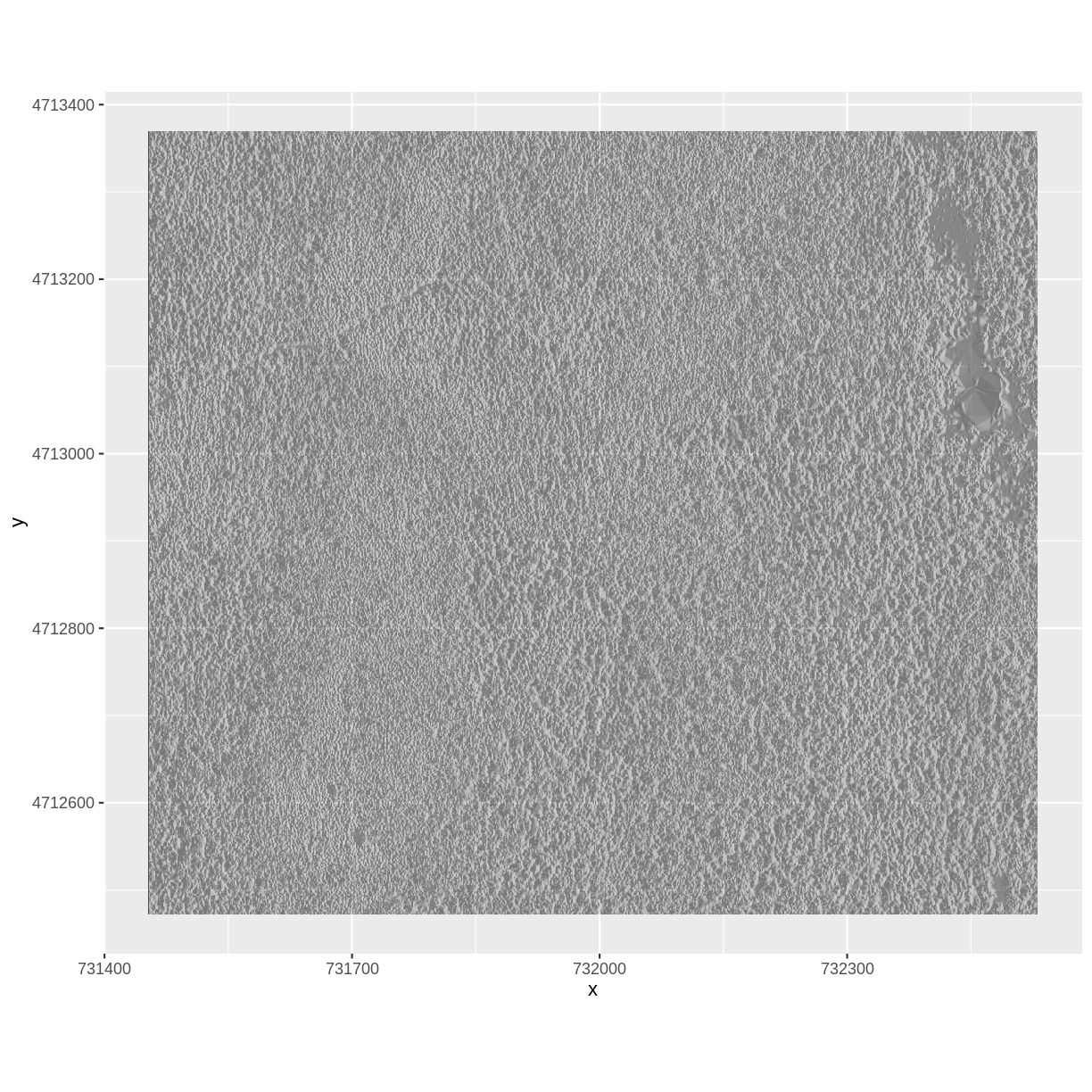## Data Tips

Turn off, or hide, the legend on a plot by adding `guide = "none"` to a `scale_something()` function or by setting `theme(legend.position = "none")`.

The alpha value determines how transparent the colors will be (0 being transparent, 1 being opaque).

We can layer another raster on top of our hillshade by adding another call to the `geom_raster()` function. Let’s overlay `DSM_HARV` on top of the `hill_HARV`.

``````ggplot() +
geom_raster(data = DSM_HARV_df ,
aes(x = x, y = y,
fill = HARV_dsmCrop)) +
geom_raster(data = DSM_hill_HARV_df,
aes(x = x, y = y,
alpha = HARV_DSMhill)) +
scale_fill_viridis_c() +
scale_alpha(range = c(0.15, 0.65), guide = "none") +
ggtitle("Elevation with hillshade") +
coord_quickmap()
``````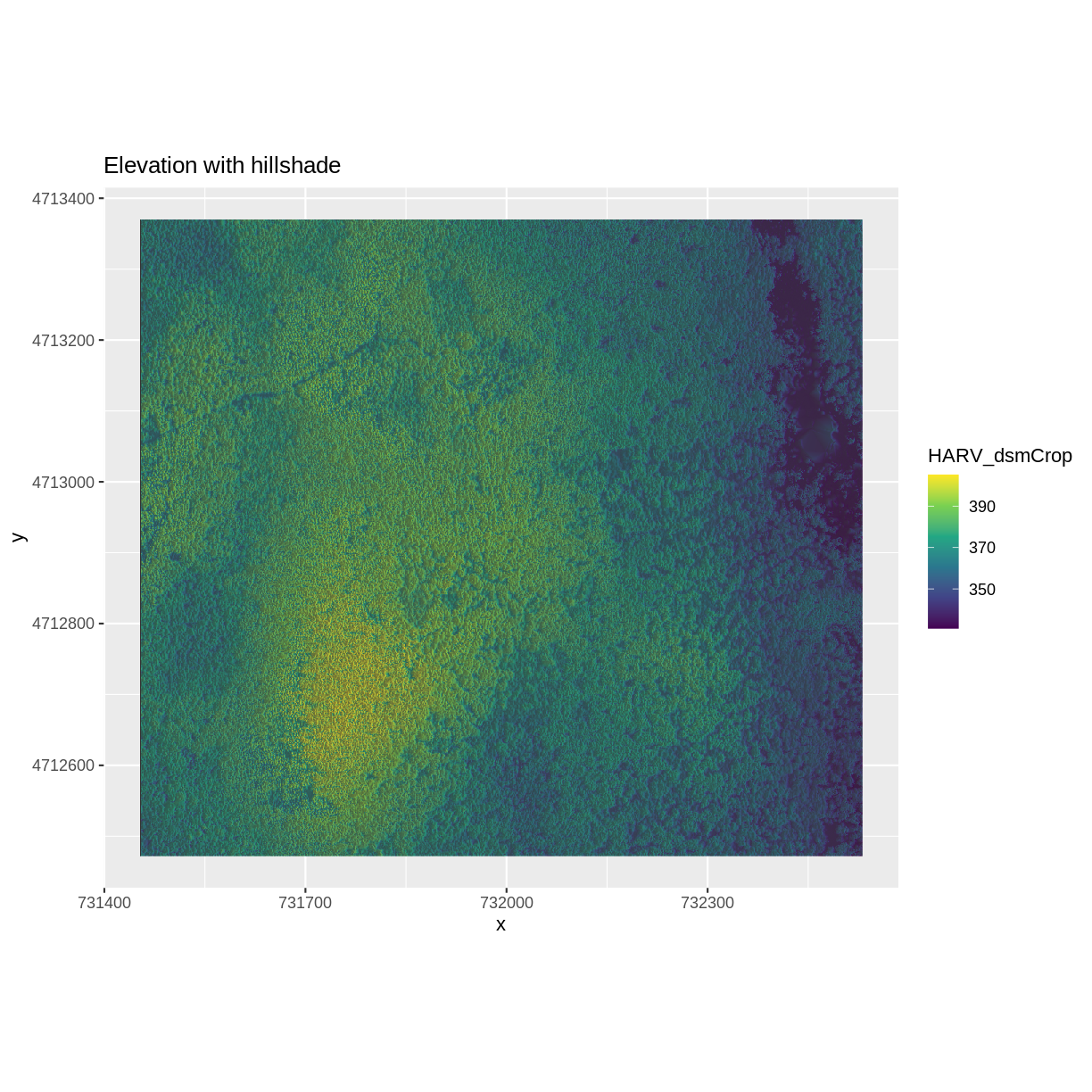## Challenge: Create DTM & DSM for SJER

Use the files in the `SJER` rastersto create a Digital Terrain Model (DTM) map of the San Joaquin Experimental Range (SJER) field site.

Make sure to:

• include hillshade in the maps,
• label axes on the DSM map and exclude them from the DTM map,
• include a title for each map,

## Answers

``````# CREATE DSM MAPS

# import DSM data
DSM_SJER <- raster("data/raster/SJER_dsmCrop.tif")
# convert to a df for plotting
DSM_SJER_df <- as.data.frame(DSM_SJER, xy = TRUE)

# import DSM hillshade
DSM_hill_SJER <- raster("data/raster/SJER_dsmHill.tif")
# convert to a df for plotting
DSM_hill_SJER_df <- as.data.frame(DSM_hill_SJER, xy = TRUE)

# Build Plot
ggplot() +
geom_raster(data = DSM_SJER_df ,
aes(x = x, y = y,
fill = SJER_dsmCrop,
alpha = 0.8)
) +
geom_raster(data = DSM_hill_SJER_df,
aes(x = x, y = y,
alpha = SJER_dsmHill)
) +
scale_fill_viridis_c() +
guides(fill = guide_colorbar()) +
scale_alpha(range = c(0.4, 0.7), guide = "none") +
xlab("UTM Easting Coordinate (m)") +
ylab("UTM Northing Coordinate (m)") +
ggtitle("DSM with Hillshade") +
coord_quickmap()
``````## Key Points

• Continuous data ranges can be grouped into categories using `mutate()` and `cut()`.

• Use built-in `terrain.colors()` or set your preferred color scheme manually.

• Layer rasters on top of one another by using the `alpha` aesthetic.